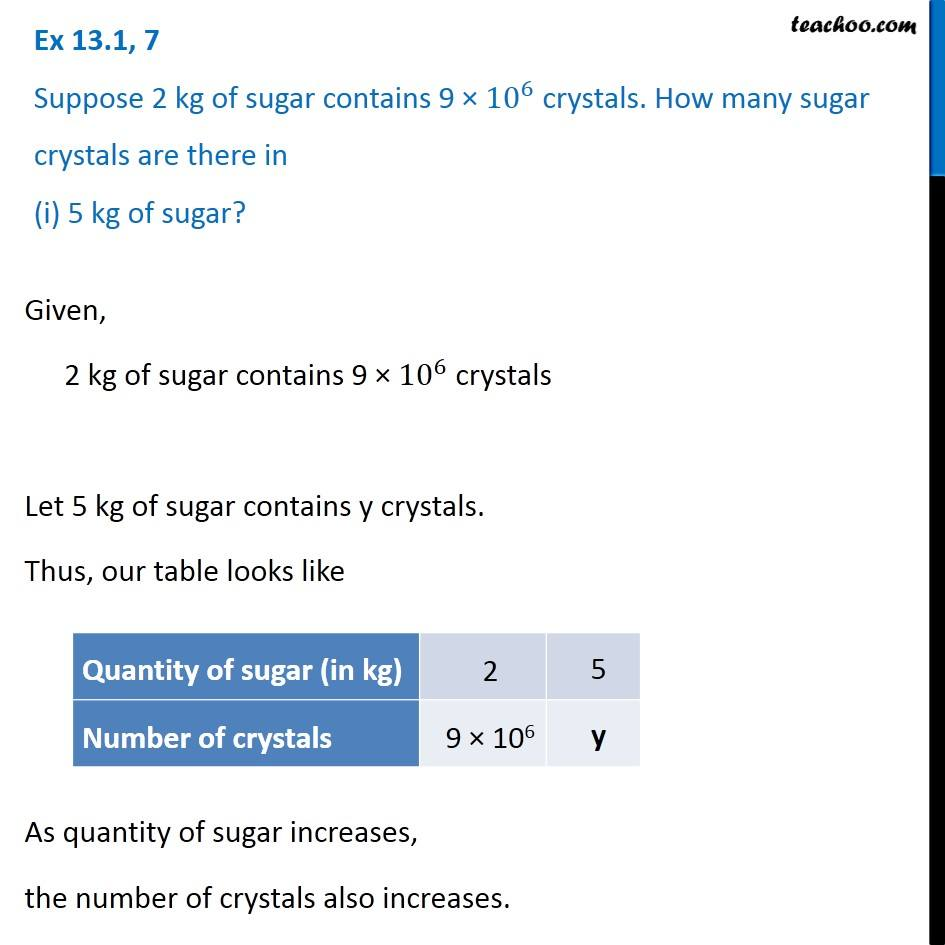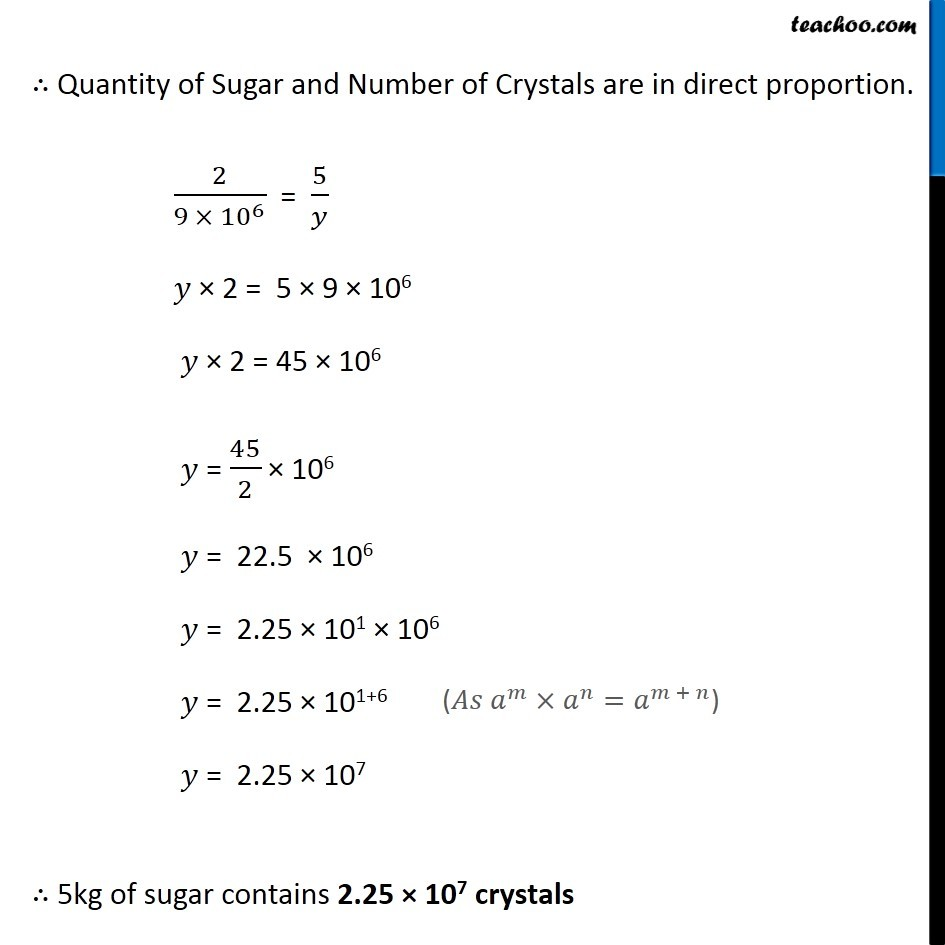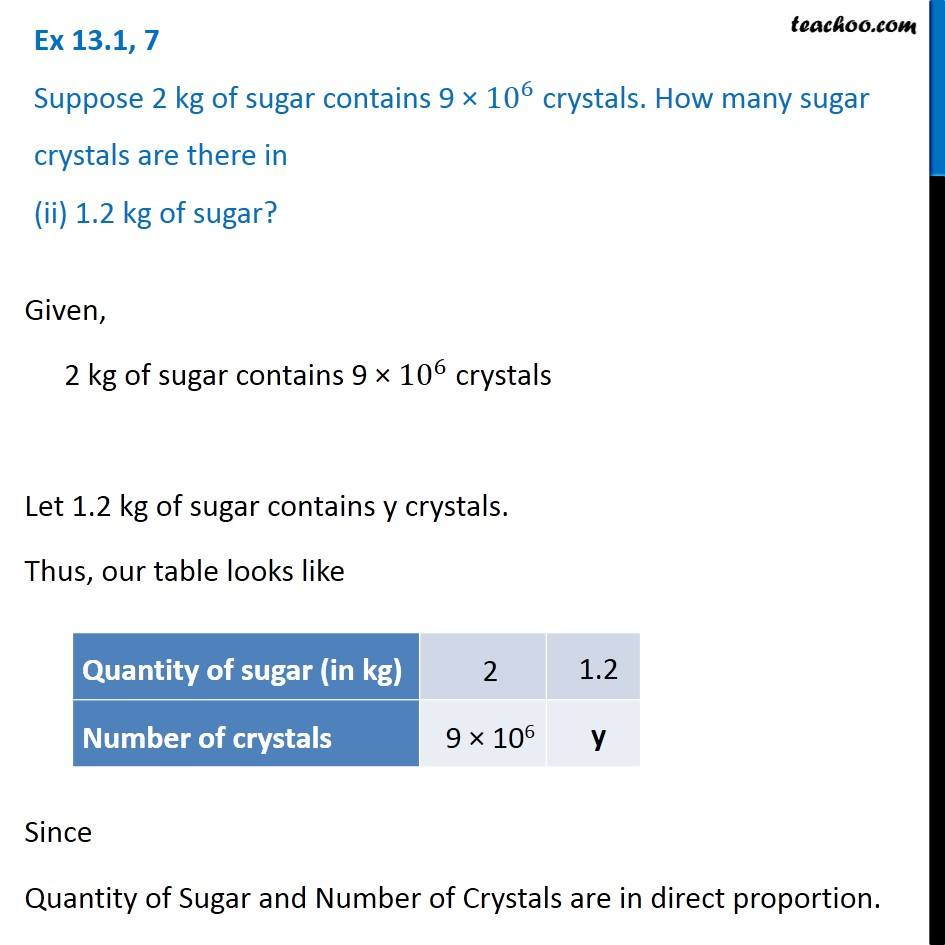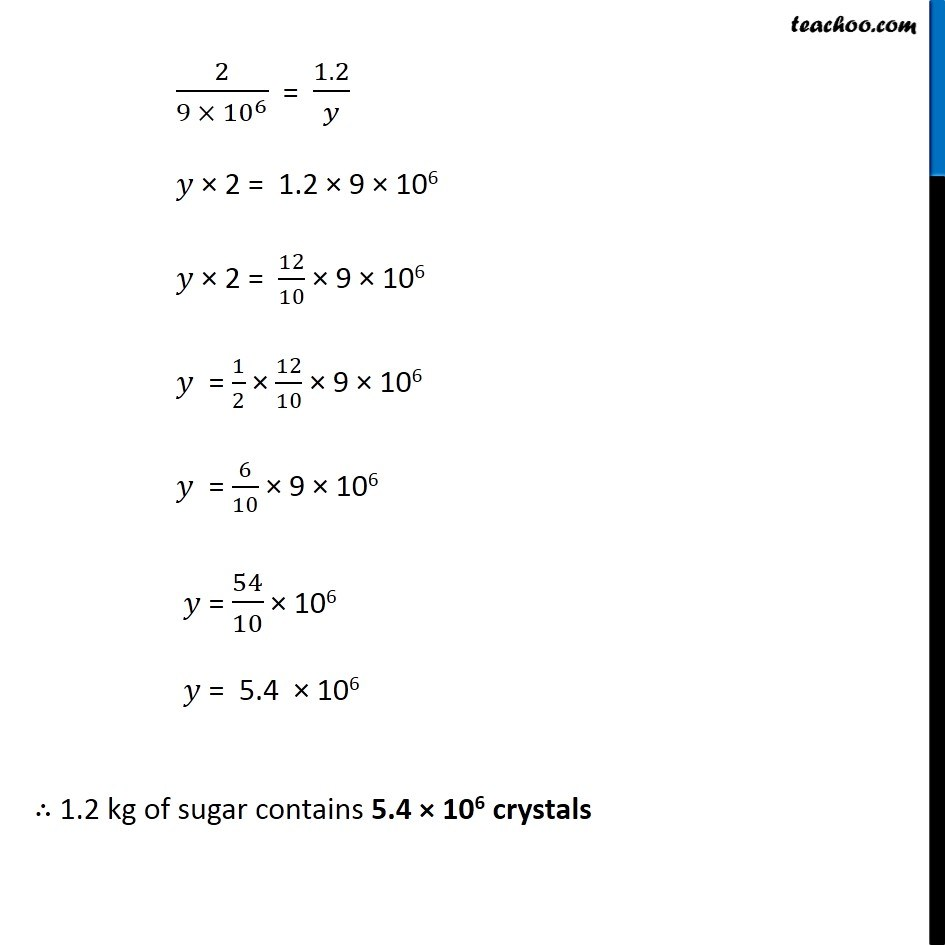1. Chapter 13 Class 8 Direct and Inverse Proportions
2. Concept wise
3. Direct Proportion

Transcript

Ex 13.1, 7 Suppose 2 kg of sugar contains 9 × 〖10〗^6 crystals. How many sugar crystals are there in (i) 5 kg of sugar? Given, 2 kg of sugar contains 9 × 〖10〗^6 crystals Let 5 kg of sugar contains y crystals. Thus, our table looks like As quantity of sugar increases, the number of crystals also increases. ∴ Quantity of Sugar and Number of Crystals are in direct proportion. 2/(9 × 〖10〗^6 ) = 5/𝑦 𝑦 × 2 = 5 × 9 × 106 𝑦 × 2 = 45 × 106 𝑦 = 45/2 × 106 𝑦 = 22.5 × 106 𝑦 = 2.25 × 101 × 106 𝑦 = 2.25 × 101+6 𝑦 = 2.25 × 107 ∴ 5kg of sugar contains 2.25 × 107 crystals Ex 13.1, 7 Suppose 2 kg of sugar contains 9 × 〖10〗^6 crystals. How many sugar crystals are there in (ii) 1.2 kg of sugar? Given, 2 kg of sugar contains 9 × 〖10〗^6 crystals Let 1.2 kg of sugar contains y crystals. Thus, our table looks like Since Quantity of Sugar and Number of Crystals are in direct proportion. 2/(9 × 〖10〗^6 ) = 1.2/𝑦 𝑦 × 2 = 1.2 × 9 × 106 𝑦 × 2 = 12/10 × 9 × 106 𝑦 = 1/2 × 12/10 × 9 × 106 𝑦 = 6/10 × 9 × 106 𝑦 = 54/10 × 106 𝑦 = 5.4 × 106 ∴ 1.2 kg of sugar contains 5.4 × 106 crystals

Direct Proportion